I.2.14

3/2/2021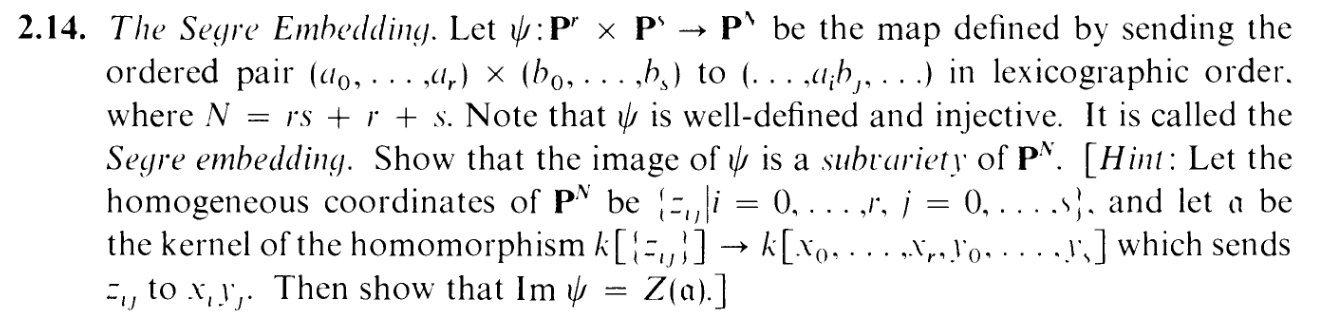Hi. This post will be quick and dry (just like sex with your wife, hahaha). Yes, an extremely scary-looking exercise. But it's actually a very similar process to what I did in
2.12b . In fact, it's even easier.

Letting θ denote the k[{zij}] k[x0,,xr,y0,,ys] map the hint gave us, we set α = ker θ and we wanna show that imϕ = Z(α).

"One inclusion is easier than the other," said 2.12b, and the statement holds analogously here. Give me a point P = (a0,,ar) × (b0,,bs) Pr × Ps, so that ϕ(P) = (,aibj,). Now give me an f(,zij,) α.

 f(…,zij,…) ∈ ker θ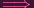θ(f(…,zij,…)) = 0f(…,θ(zij),…) = 0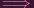f(…,xiyj,…) = 0f(…,ai,bj,…) = 0f(ϕ(P)) = 0

Since f α was arbitrary, ϕ(P) Z(α). And since P was arbitrary, imϕ Z(α)

Now for the reverse inclusion. Given C = (,cij,) Z(α), can we find P = (a0,,ar) × (b0,,bs) Pr ×Ps such that ϕ(P) = C

I.e. we need to satisfy (,aibj,) = (,cij,).

I.e. we need to satisfy aibj = cij (up to a constant multiple independent of i,j).

So what do we do? Same thing in 2.12b: Line up the requirements, "force" values for our ai,bjs, verify that they actually work.

First, I'll assume that c000 without loss of generality. Now, let a0 be.... anything but zero. Yes. You heard me right. Let a0 be literally fucking anything you want it to be, reader, as long as it's nonzero and from k.

Then check out these requirements:

 a0b0 = c00 a0b0 = c00 a0b1 = c01 a1b0 = c10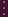a0bj = c0j aib0 = ci0a0bs = c0s arb0 = cr0

As soon as a0 is fixed, so is everything else:

 b0 = c00∕a0 a0 = c00∕b0 b1 = c01∕a0 a1 = c10∕b0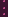bj = c0j∕a0 ai = ci0∕b0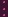bs = c0s∕a0 ar = cr0∕b0

So choosing anything for a0 forces all of P. Now we just have to check that ϕ(P) does indeed equal C. I.e. we need to check if aibj = cij. Well, based on our settings,

 aibj =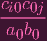=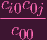Now suppose, for the sake of contradiction,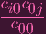did NOT equal cij. Then that would mean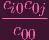≠cij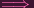ci0c0j ≠cijc00ci0c0j - cijc00 ≠ 0

I.e. we'd have that C does NOT satisfy the polynomial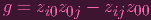But, of course,

 θ(g) = xiy0x0yj - xiyjx0y0 = 0

So g ker θ i.e. g α. By assumption, C Z(α), yielding a contradiction.

Don't worry. The next exercise is another 3-parter.